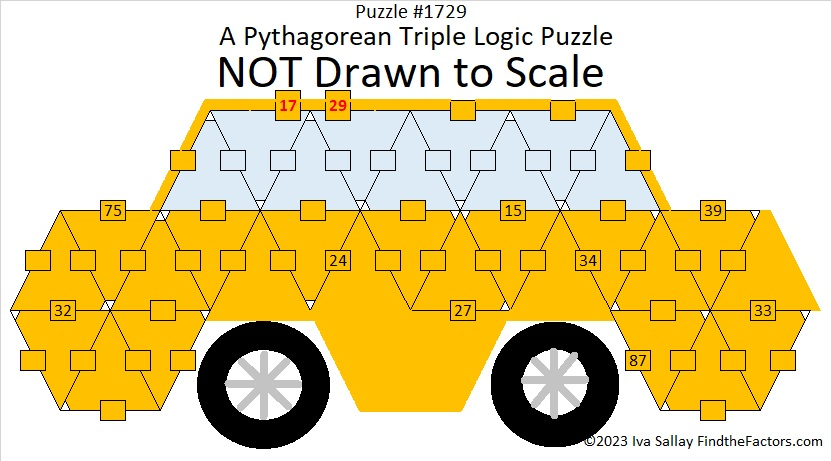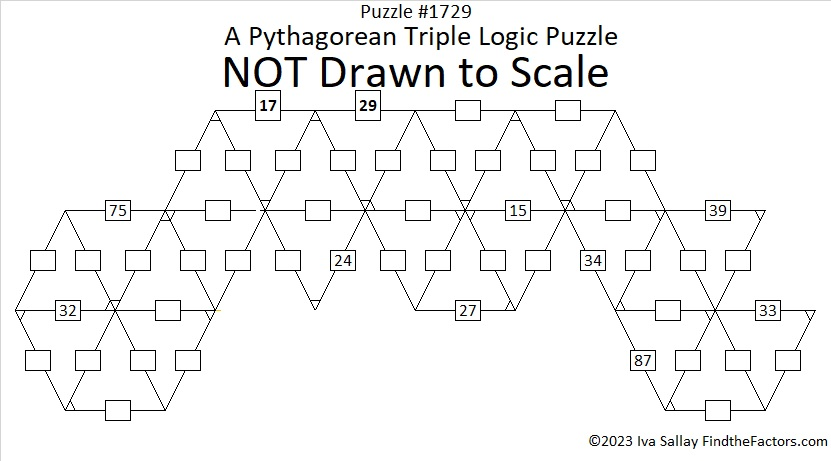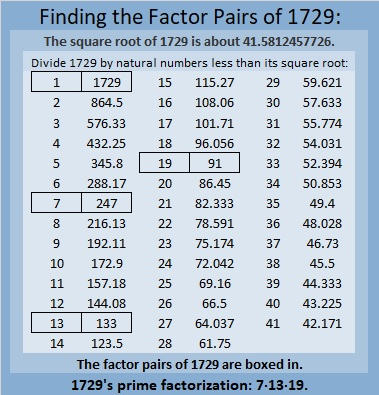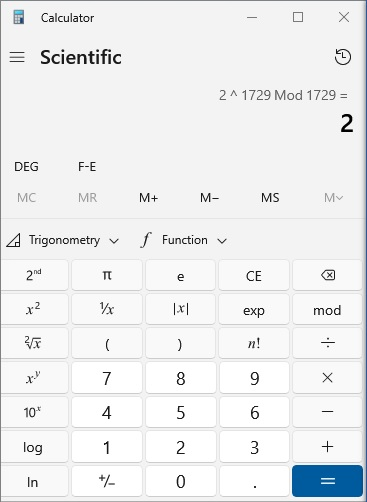# 1729 Not a Dull Number

### Today’s Puzzle:

A little more than a hundred years ago near Cambridge University G. H. Hardy took a taxi to visit his young friend and fellow mathematician, Srinivasa Ramanujan, in the hospital. Hardy couldn’t think of anything interesting about his taxi number, 1729, and remarked to Ramanujan that it appeared to be a rather dull number. But even the reason for his hospitalization could not prevent Ramanujan’s genius from shining through. He immediately recognized 1729’s unique and very interesting attribute: it is the SMALLEST number that can be written as the sum of two cubes in two different ways! Indeed,
12³ + 1³ = 1729, and
10³ + 9³ = 1729.

Today’s puzzle looks a little bit like a modern-day American taxi cab with the clues 17 and 29 at the top of the cab. The table below the puzzle contains all the Pythagorean triples with hypotenuses less than 100 sorted by legs and by hypotenuses. Use the table and logic to write the missing sides of the triangles in the puzzle. The right angle on each triangle is the only one that is marked. Obviously, none of the triangles are drawn to scale. No Pythagorean triple will appear more than once in the puzzle.Here’s the same puzzle without all the added color:Print the puzzles or type the solutions in this excel file: 10 Factors 1721-1729.

What taxi cab might Hardy have tried to catch next? He might have had to wait a long time for it, 4104.
16³ + 2³ = 4104, and
15³ + 9³ = 4104.

### Factors of 1729:

• 1729 is a composite number.
• Prime factorization: 1729 = 7 × 13 × 19.
• 1729 has no exponents greater than 1 in its prime factorization, so √1729 cannot be simplified.
• The exponents in the prime factorization are 1, 1, and 1. Adding one to each exponent and multiplying we get (1 + 1)(1 + 1)(1 + 1) = 2 × 2 × 2 = 8. Therefore 1729 has exactly 8 factors.
• The factors of 1729 are outlined with their factor pair partners in the graphic below.### More About the Number 1729:

Did you notice these cool-looking factor pairs?
19 · 91 = 1729.
13 · 133 = 1729.

1729 is the hypotenuse of a Pythagorean triple:
665-1596-1729 which is 133 times (5-12-13).

1729 is the difference of two squares in four different ways:
865² – 864² = 1729,
127² – 120² = 1729,
73² – 60² = 1729, and
55² – 36² = 1729.

1729 is also a pseudoprime number! For example, even though it is a composite number, it passes the quick prime number test I first wrote about in 341 is the smallest composite number that gives a false positive for this Quick Prime Number Test:It is known as a Carmichael number because back in 1909, Robert D. Carmichael, an American mathematician, showed that it passed even more prime number tests than this one.